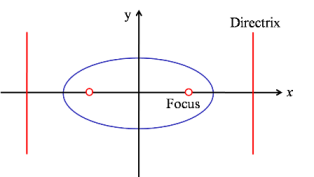The Ellipse is a plane curve. The Ellipse is a conic section.

The Ellipse is defined as the path of a point (locus) which moves such that its distance from a fixed point (called the focus) is less than its distance from a fixed line (called the directrix).

The ratio of the distance to the focus to the distance to the directrix is called the eccentricity normally denoted by e.

For an ellipse therefore e < 1.

When the directrix is $x=\frac{a}{e}$ and the focus is (ae,0) the ellipse will have the equation in Cartesian Coordinates $\frac{x^2}{a^2}+\frac{y^2}{b^2}=1$ where $b^2=a^2(1-e^2)$

Due to symmetry each ellipse will have 2 foci. An interesting property of the ellipse is that the sum of the distances from any point on the ellipse to the foci is constant. A consequence of this is that you can draw an ellipse by putting two pins into a surface, dropping a loop of string over them and a pencil, then drawing while holding the string taut.

• You can find an animated example here:
Kepler showed that orbits of the planets are better approximated by ellipses, with the Sun at one focus, than by circles.
One of the Named Curves on this site.